• 参数估计主要目的是借助总体X一个样本来计算总体未知参数近似值，即在已知总体X分布函数形式情况下，同样本来估计未知参数值。 1、最大似然估计法 假设已知总体X分布律P={X=x}=p(x;θ)p(x;\theta)...
参数估计主要目的是借助总体X的一个样本来计算总体未知参数的近似值，即在已知总体X的分布函数的形式的情况下，同样本来估计未知参数的值。
1、最大似然估计法
假设已知总体X的分布律P={X=x}=p(x;θ)<!--//--><![CDATA[//><!--
p(x;\theta)
//--><!]]>，X1<!--//--><![CDATA[//><!--
X_1
//--><!]]>,X2<!--//--><![CDATA[//><!--
X_2
//--><!]]>,…,Xn<!--//--><![CDATA[//><!--
X_n
//--><!]]>是来自X的n个样本，x1<!--//--><![CDATA[//><!--
x_1
//--><!]]>,x2<!--//--><![CDATA[//><!--
x_2
//--><!]]>,…,xn<!--//--><![CDATA[//><!--
x_n
//--><!]]>是样本相应的一个样本值，则X1<!--//--><![CDATA[//><!--
X_1
//--><!]]>,X2<!--//--><![CDATA[//><!--
X_2
//--><!]]>,…,Xn<!--//--><![CDATA[//><!--
X_n
//--><!]]>的联合分布律，即事件{X1=x1,X2=x2,...,Xn=xn}<!--//--><![CDATA[//><!--
\{X_1=x_1,X_2=x_2,...,X_n=x_n\}
//--><!]]>发生的概率为

L(θ)=L(x1,x2,...,xn;θ)=∏ni=1p(xi;θ)<!--//--><![CDATA[//><!--
L(\theta)=L(x_1,x_2,...,x_n;\theta)=\prod_{i=1}^np(x_i;\theta)
//--><!]]>

L(θ)<!--//--><![CDATA[//><!--
L(\theta)
//--><!]]>称为样本的似然函数。参数θ<!--//--><![CDATA[//><!--
\theta
//--><!]]>的最大似然估计值为θ‘<!--//--><![CDATA[//><!--
\theta
//--><!]]>的值使得L(θ)<!--//--><![CDATA[//><!--
L(\theta)
//--><!]]>的取值最大，即 L(x1,x2,...,xn;θ‘)=maxL(x1,x2,...,xn;θ)<!--//--><![CDATA[//><!--
L(x_1,x_2,...,x_n;\theta)=maxL(x_1,x_2,...,x_n;\theta)
//--><!]]>
而相应的统计量θ‘(X1,X2,...,Xn)<!--//--><![CDATA[//><!--
\theta(X_1,X_2,...,Xn)
//--><!]]>称为参数θ<!--//--><![CDATA[//><!--
\theta
//--><!]]>的最大似然估计量。
展开全文最大似然估计
• DSP芯片，也称数字信号处理器，是一...　水声信号处理的主要目的 ,一是对接收信号通过一定的处理 ,进行特征提取与分析识别 ,进而对目标进行判断、分类 ;二是对信号的特征参数进行运算处理 ,给出目标的状态参量。目前普
• Data2Dynamics软件 联系方式：安德烈亚斯Raue - （支持问题，请使用问题和论坛，谢谢！... 关键特征：可靠高效的参数估计技术以及对参数，测量和预测不确定性统计评估。 一些特殊功能： 该框架可以
• 本文的主要目的是利用归约技术研究参数估计方法的行为和性质。 我们将重点介绍一种使用修正得分函数来减少最大似然估计值偏差的方法。 我们还通过不同样本量和结果变量概率百分比的模拟数据提供了有趣的新示例。
• 本文的主要目的是设计一个估计器，以便对于所有可能的量化误差和数据包丢失，状态估计误差序列是收敛的。 通过选择随机成本函数，可以通过求解带有不确定参数的正则化最小二乘问题来获得最佳估计量。 所提出的方法...
• 主要目的是通过提取空间中按一定方式排列传感器阵列所接收信号有用特征，用来估计信号空域方位参数，因此国内外学者提出了一系列DOA估计新算法，其中基于子空间分解多种信号分类（Multiple Signal ...
• 这样做的主要目的是找出在使用LINEX损失函数时哪种重采样方法效果良好。 使用蒙特卡洛模拟，比较这些估计量。 它从伽马分布中提取随机数，找到θis的最大似然估计，并用该估计器解释LINEX损失函数; 或，其中c是形状...
• 原创文章,如需转载请保留出处 ...我们现在的主要目的是求1式的最大值,其中1式通过贝叶斯公式能转换为2式的形式,其中2式的分母相同,我们只需要考虑分子即可–即3式,又因为P(Ai)近似相等.所以我们可以将3式...
原创文章,如需转载请保留出处
本博客为七月在线邹博老师机器学习数学课程学习笔记

一. 极大似然估计
1.1 极大似然估计
假设D是样本集合,A是参数集合,我们在D样本集固定的情况下,A取何值时,P(A|D)能取到最大。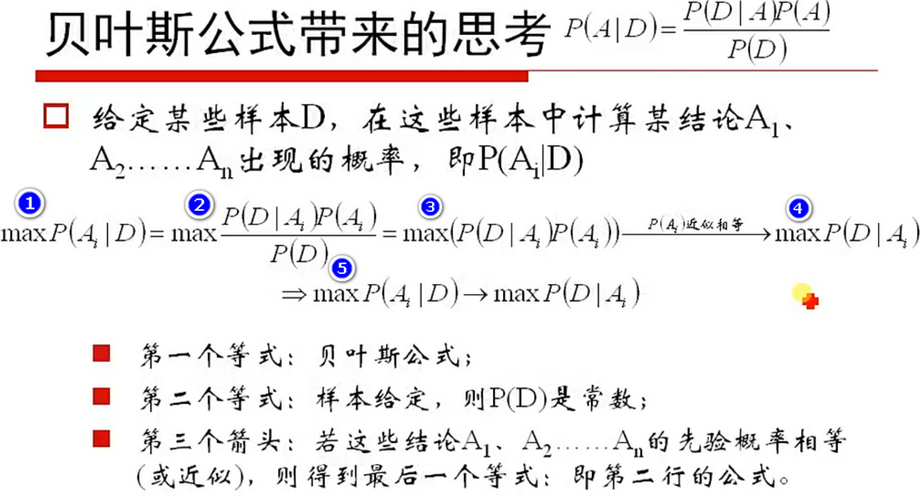我们现在的主要目的是求1式的最大值,其中1式通过贝叶斯公式能转换为2式的形式,其中2式的分母相同,我们只需要考虑分子即可–即3式,又因为P(Ai)近似相等.所以我们可以将3式中P(Ai)去掉,直接考虑4式的最大值.
最后我们得到5式,这相当于是一种颠倒原因结果的式子。

1.2 极大似然估计定义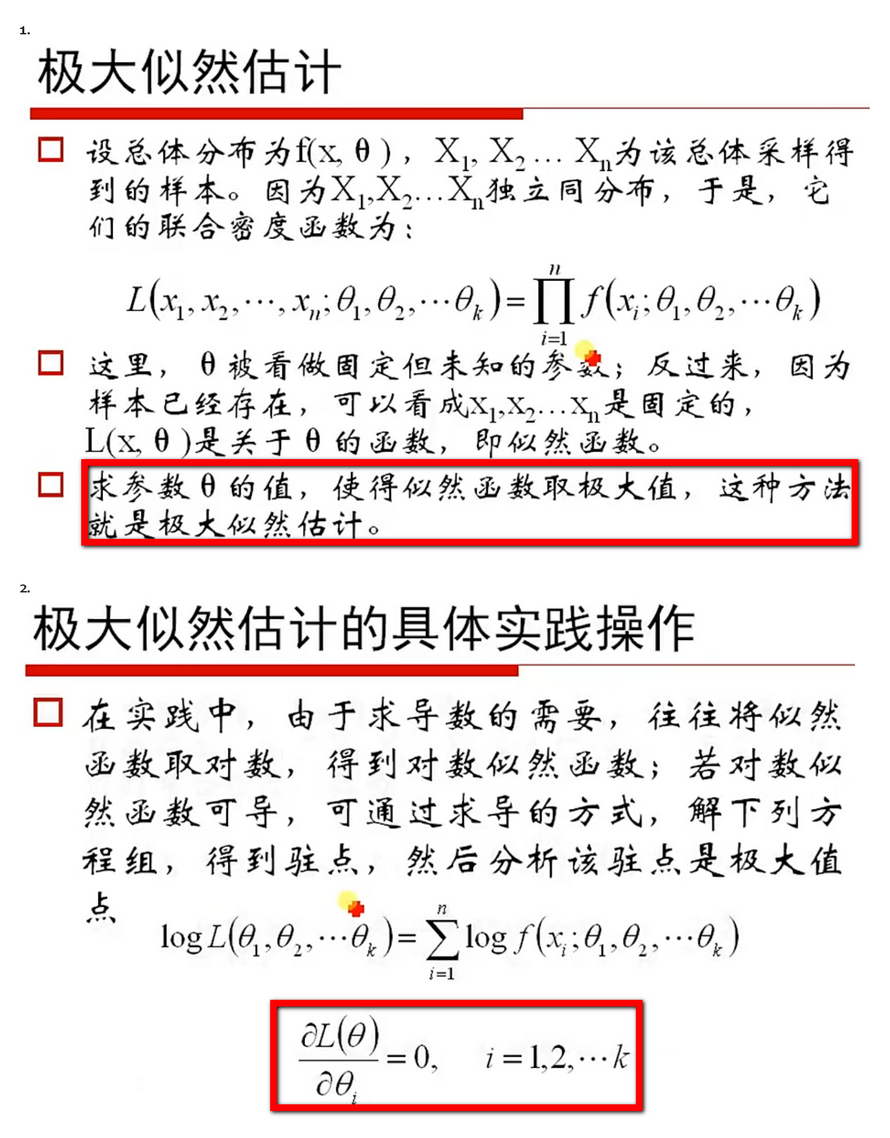1.3 二项分布中的极大似然估计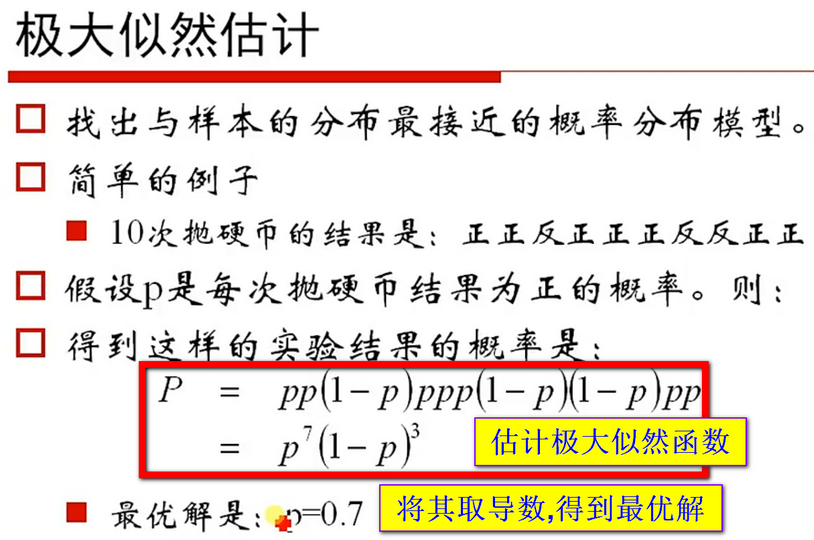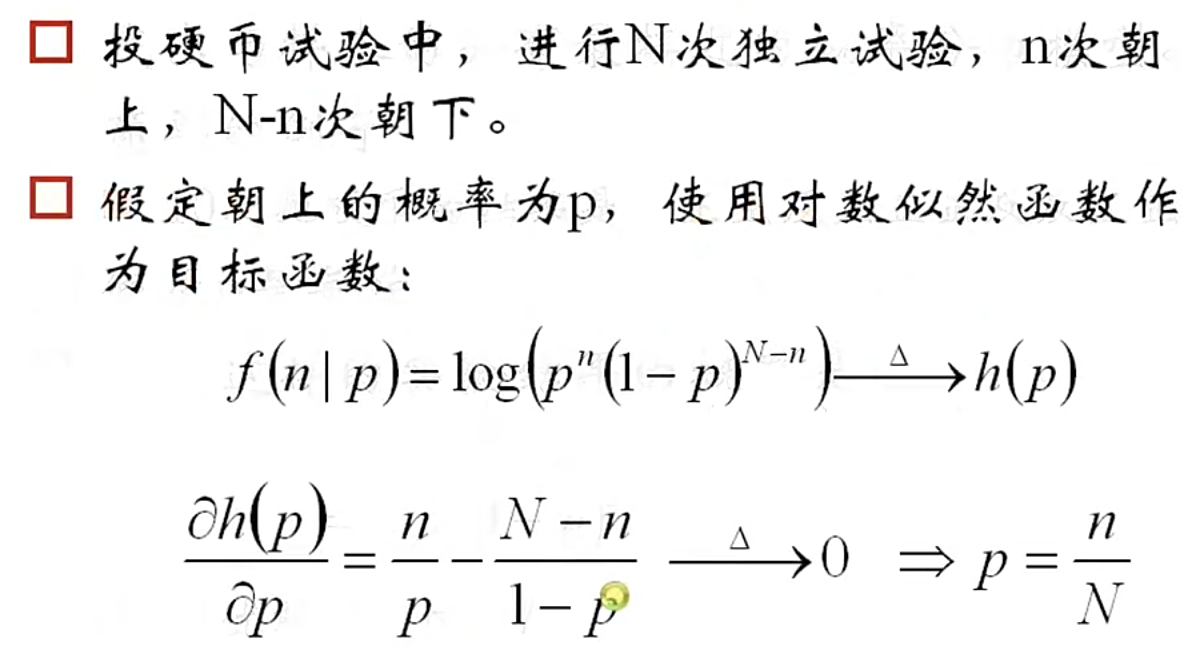1.4 高斯分布中的极大似然估计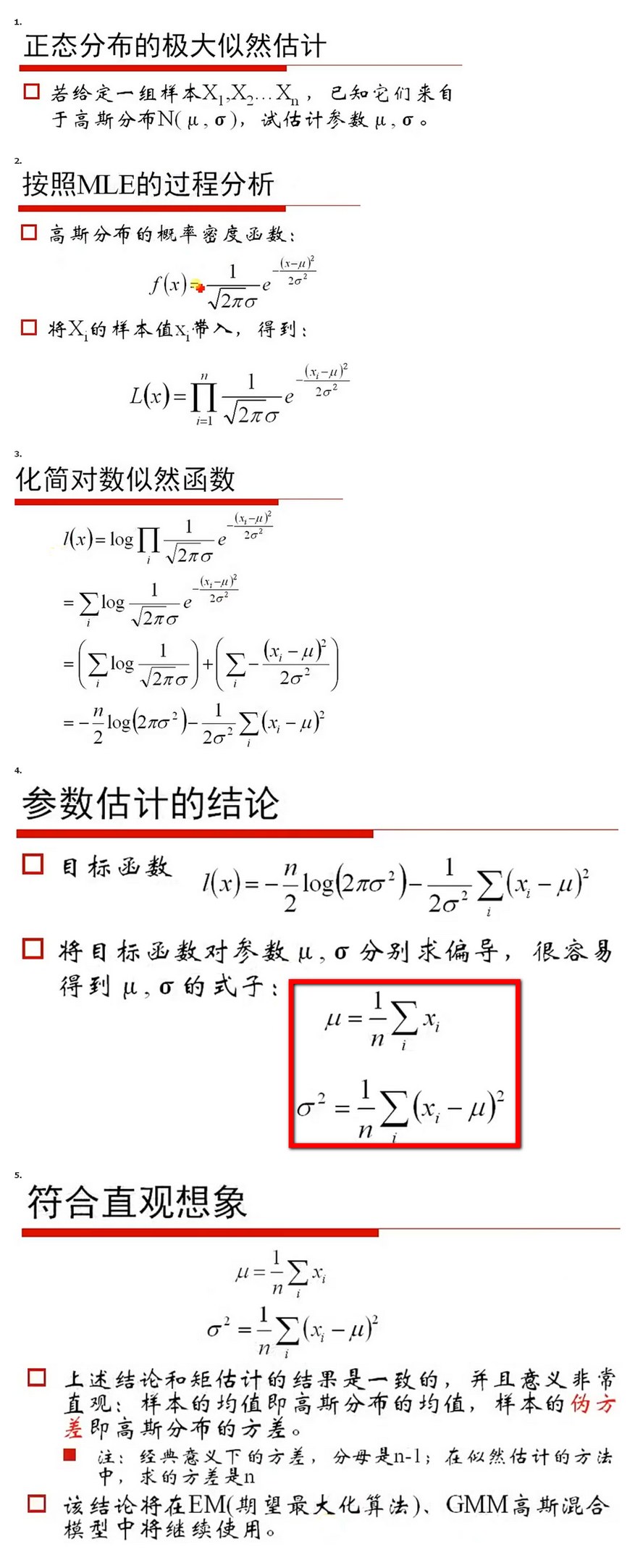1.5 极大似然估计与过拟合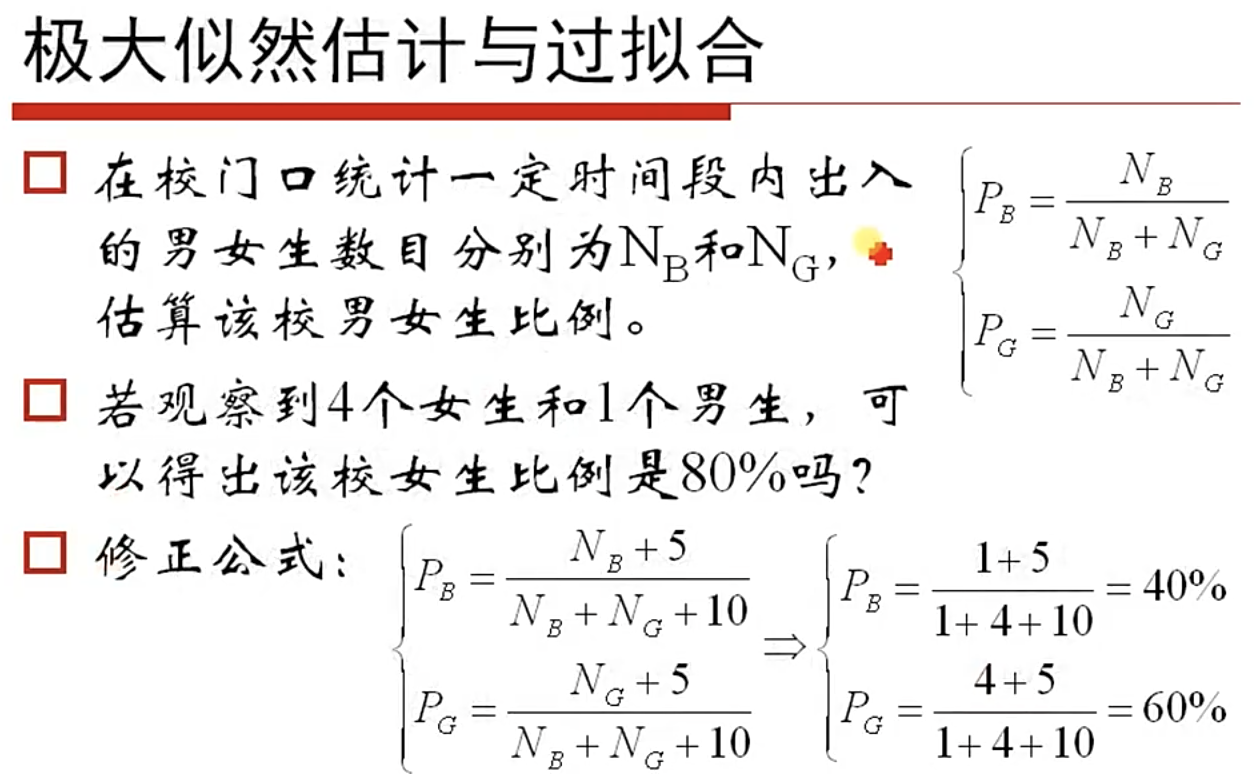展开全文机器学习
• DOA估计是雷达参数估计方面理论研究一个重要内容，其主要目的是确定各个信号到达阵列参考阵元方向角。MIMO雷达可以有效提高DOA估计分辨力和精度得到了广泛关注，但是MIMO雷达DOA估计算法却比较复杂。因此对...
• ## IRT模型估计-EM算法

千次阅读 2019-05-22 09:12:28
主要目的是通过被试(examinees) 对于一套试题反应(responses), 对被试 能力(ability parameters) 参数θ\thetaθ 和题目(item parameters) 作出估计。由于被试反应变量一般是离散变量，基本是0-1反应变量....
IRT模型中参数估计
IRT(Item Response Theory) 项目反应理论。是教育评估与心理测量理论中的重要模型。主要目的是通过被试(examinees) 的对于一套试题的反应(responses), 对被试 的能力(ability parameters) 参数$\theta$ 和题目(item parameters) 作出估计。由于被试的反应变量一般是离散变量，基本的是0-1反应变量，link 函数一般有probit和logit两种。例如，2pl, 2pno,3pl等。
而对于不同的反应模型，估计方法大致相同，总体来说分为两大体系。频率框架下是联合极大似然估计(Joint Maximum Likelihood Estimation, JMLE) 和边际极大似然(Marginal Maximum Likelihood Estimation, MMLE). Bayesian 框架下，常用估计方法有Gibbs算法、MCMC算法及其衍生估计方法。
下面要介绍的EM算法是基于logit link下的2pl模型进行EM估计。
2pl模型的EM估计

2pl模型
2pl模型，全称是two parameter logistic model，实际上是logistics 回归中的一种。
假设$i=1,2,……,N$ 个人作答了$j=1,2,……,J$ 个题目，相应的反应矩阵$Y=(Y_{ij})_{i=1,j=1}^{N, J}$ 代表第$i$ 个人在作答第$j$ 题的反应，也就是说，$Y_{ij}=1$ 代表答对了，否则代表没有答对，这里只考虑两值反应。假设人的能力参数$\theta_{i}$，项目参数集合 $\varOmega=\left\lbrace \boldsymbol{a}=(a_{j})_{j=1}^{J}, \boldsymbol{d}=(d_{j})_{j=1}^{J}\right\rbrace$， 表示项目参数区分度a和难度参数的等价参数d。
2pl模型的项目反应函数如下，
$L(Y;\boldsymbol{\theta}, \boldsymbol{a}, \boldsymbol{d})=\prod_{i=1}^{N} \prod_{j=1}^{J} P(Y_{ij}=1;\theta_{i}, a_{j}, d_{j})^{Y_{ij}}P(Y_{ij}=0;\theta_{i}, a_{j}, d_{j})^{1-Y_{ij}}$
其中，
$P(Y_{ij}=1;\theta_{i}, a_{j}, d_{j})=\frac{exp(a_{j}\theta_{i}+d_{j})}{1+exp(a_{j}\theta_{i}+d_{j})}$
Note, 这个似然也可以写成是
$L(Y;\boldsymbol{\theta}, \boldsymbol{a}, \boldsymbol{d})=\prod_{i=1}^{N} \prod_{j=1}^{J}\frac{exp(y_{ij}(a_{j}\theta_{i}+d_{j}))}{1+exp(a_{j}\theta_{i}+d_{j})}$

EM估计具体计算过程
EM算法，也叫边际极大似然估计，marginal maximum likelihood estimation。基本想法就是把潜在变量(latent variable) $\theta$ 积分掉之后的边际似然函数，求极大似然估计。
2pl的对数边际似然函数为，
$logL(Y;\boldsymbol{a}, \boldsymbol{d})=log\int _{\boldsymbol{\theta}}L(Y;\boldsymbol{\theta}, \boldsymbol{a}, \boldsymbol{d})g(\boldsymbol{\theta})d\boldsymbol{\theta}$
$=\sum_{i=1}^{N}log\int\prod_{j=1}^{J}\frac{exp(y_{ij}(a_{j}\theta_{i}+d_{j}))}{1+exp(a_{j}\theta_{i}+d_{j})}g(\theta_{i})d\theta_{i}$
其中$g(\boldsymbol{\theta})$ 是能力参数$\boldsymbol{\theta}$ 的先验分布。目标是，求$(\widehat \boldsymbol{a}, \widehat \boldsymbol{d})=argmax \left\lbrace logL(Y;\boldsymbol{a}, \boldsymbol{d})\right\rbrace.$对于这种最优化问题，一般常用的方法常用的方法有Newton，GD等方法。Newton方法是需要解方程$0=\frac{\partial logL(Y;\boldsymbol{a}, \boldsymbol{d})}{\partial a_{j}}$，除了一阶导数之外，还需要再求二阶导数。GD，梯度下降方法，只需要一阶导数，具体原理见下面wiki百科。
综上，一阶导数是必须要求的，然后上面的式子对$a_{j}$求一阶偏导，
$\frac{\partial logL(Y;\boldsymbol{a}, \boldsymbol{d})}{\partial a_{j}}=\frac{\partial}{\partial a_{j}}log \int _{\boldsymbol{\theta}}L(Y;\boldsymbol{\theta}, \boldsymbol{a}, \boldsymbol{d})g(\boldsymbol{\theta})d\boldsymbol{\theta}$

$=\frac{1}{\int _{\boldsymbol{\theta}}L(Y;\boldsymbol{\theta}, \boldsymbol{a}, \boldsymbol{d})g(\boldsymbol{\theta})d\boldsymbol{\theta}}\int _{\boldsymbol{\theta}}\frac{\partial}{\partial a_{j}}L(Y;\boldsymbol{\theta}, \boldsymbol{a}, \boldsymbol{d})g(\boldsymbol{\theta})d\boldsymbol{\theta}$
$= \int_{\boldsymbol{\theta}} \frac{L(Y;\boldsymbol{\theta}, \boldsymbol{a}, \boldsymbol{d})g(\boldsymbol{\theta})}{\int _{\boldsymbol{\theta}}L(Y;\boldsymbol{\theta}, \boldsymbol{a}, \boldsymbol{d})g(\boldsymbol{\theta})d\boldsymbol{\theta}}\frac{\partial}{\partial a_{j}}logL(Y;\boldsymbol{\theta}, \boldsymbol{a}, \boldsymbol{d})d\boldsymbol{\theta},$
$=\sum_{i=1}^{N}\int_{\theta_{i}} (y_{ij}-\frac{exp(a_{j}\theta_{i}+d_{j})}{1+exp(a_{j}\theta_{i}+d_{j})})\theta_{i}P(\theta_{i}|Y_{i.},\boldsymbol{a},\boldsymbol{d})d{\theta_{i}}.$
因为$\frac{L(Y;\boldsymbol{\theta}, \boldsymbol{a}, \boldsymbol{d})g(\theta)}{\int _{\boldsymbol{\theta}}L(Y;\boldsymbol{\theta}, \boldsymbol{a}, \boldsymbol{d})g(\theta)d\boldsymbol{\theta}}=P(\boldsymbol{\theta}|Y,\boldsymbol{a},\boldsymbol{d})$ 是$\theta$的联合后验分布，同时
$\frac{\partial}{\partial a_{j}}logL(Y;\boldsymbol{\theta}, \boldsymbol{a}, \boldsymbol{d})=\frac{\partial}{\partial a_{j}}\sum_{i=1}^{N}\sum_{j=1}^{J}y_{ij}(a_{j}\theta_{i}+d_{j})-log(1+exp(a_{j}\theta_{i}+d_{j}))$
$=\sum_{i=1}^{N}(y_{ij}-\frac{exp(a_{j}\theta_{i}+d_{j})}{1+exp(a_{j}\theta_{i}+d_{j})})\theta_{i}.$
但是实际上，由于积分对于计算机来说是很庞大的计算量，所以在计算过程中，一般使用有限的节点的离散分布来逼近这个连续积分。具体做法如下，对所有人的$\theta_{i}$取区间[-4,4] 内的节点$X_{ik}, k=1,2,……,q$。假设先验密度服从标准正态分布，对于第$i$个人的后验密度函数为，
$P(\theta_{i}=X_{ik}|Y_{i.},\boldsymbol{a},\boldsymbol{d})=\frac{\frac{exp(\sum_{j=1}^{J}y_{ij}(a_{j}X_{ik}+d_{j})-\frac{X_{ik}^{2}}{2})}{\prod_{j=1}^{J}(1+exp(a_{j}X_{ik}+d_{j}))}}{\sum_{k=1}^{q}\frac{exp(\sum_{j=1}^{J}y_{ij}(a_{j}X_{ik}+d_{j})-\frac{X_{ik}^{2}}{2})}{\prod_{j=1}^{J}(1+exp(a_{j}X_{ik}+d_{j}))}}.$
从而，
$\frac{\partial logL(Y;\boldsymbol{a}, \boldsymbol{d})}{\partial a_{j}}=\sum_{k=1}^{q}\sum_{i=1}^{N}(y_{ij}-\frac{exp(a_{j}X_{ik}+d_{j})}{1+exp(a_{j}X_{ik}+d_{j})})X_{ik}P(\theta_{i}=X_{ik}|Y_{i.},\boldsymbol{a},\boldsymbol{d}).$
以上这个方程就是在EM算法当中目标要求的边际似然函数，剩下的是利用最优化的方法来得到最优解。参数$\boldsymbol{d}$ 也是类似得到一阶导数。

具体的GD算法步骤如下，
1，initial values $(\boldsymbol{a}^{(0)},\boldsymbol{d}^{(0)})$;
2，posterior density $P(\theta_{i}=X_{ik}|Y_{i.},\boldsymbol{a},\boldsymbol{d})$;
3，gradient $\frac{\partial logL(Y;\boldsymbol{a}, \boldsymbol{d})}{\partial a_{j}}|_{(a_{j}^{(0)},d_{j}^{(0)})}$ and $\frac{\partial logL(Y;\boldsymbol{a}, \boldsymbol{d})}{\partial d_{j}}|_{(a_{j}^{(0)},d_{j}^{(0)})}$;
4，update $(a_{j},d_{j})$ by
$a_{j}^{(1)}=a_{j}^{(0)}+stepsize*\frac{\partial logL(Y;\boldsymbol{a}, \boldsymbol{d})}{\partial _{j}}|_{(a_{j}^{(0)},d_{j}^{(0)})}\\d_{j}^{(1)}=d_{j}^{(0)}+stepsize *\frac{\partial logL(Y;\boldsymbol{a}, \boldsymbol{d})}{\partial d_{j}}|_{(a_{j}^{(0)},d_{j}^{(0)})};$
5，repeat until the tolerance, that is, the difference of the k$th$ and (k+1)$th$ step is under some values or satisfying other criteriors.
模拟研究

生成模拟数据
假设$N=2000$, $J=20$, $a\sim runif(J,0,2)$, $d\sim runif(J,-1,1)$, $\theta_{i}\sim rnorm(1,0,1)$。根据2pl模型生成数据，下面是R的code。

N <- 2000;J <- 20;
theta_true <- cbind(rep(1,N),rnorm(N));
a_true<-runif(J,0,2);d_true<-runif(J,-1,1);A_true<-cbind(d_true,a_true);
P_true<-1/(1+exp(-theta_true%*%t(A_true)));
response <- matrix(rbinom(N*J,1,P_true),nrow=N,ncol=J);#response
colnames(response)<-1:J;

以下是反应矩阵的前5行，也就是前5个人的20题的反应向量。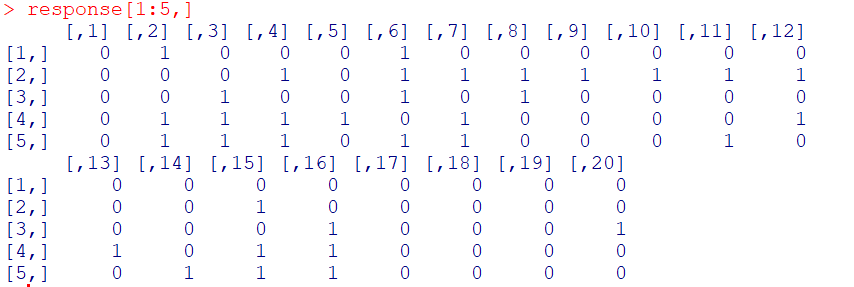估计过程。
–R package “mirt”.
在R package “mirt” 中，提供了已有的函数mirt直接进行估计，详细的R包的introduction等见下面的链接和参考文献。以下是代码。

library(mirt);
aa<-mirt(response,1,verbose=FALSE);
bb <- cbind(A_true,coef(aa,simplify=TRUE)[][,2],coef(aa,simplify=TRUE)[][,1])[,1:4];
colnames(bb)<-c("d_true","a_true","d_estimator","a_estimator");

以下是输出结果，结果的average bias差不多是0.02503983。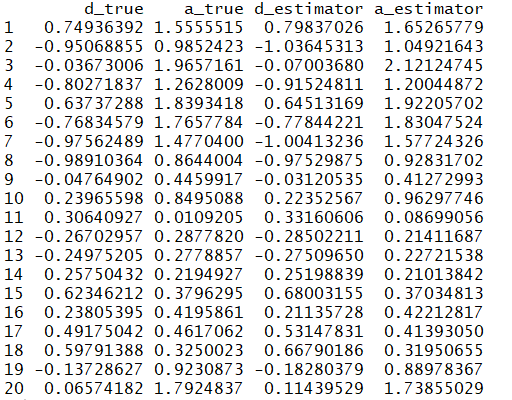–GD方法，自己编的code，如下。
#####my code####
#initial value
d_initial <- d_0 <- runif(J,-1,1);a_initial <- a_0 <- runif(J,0,2)
THETA_TUTA <- seq(-4,4,by=8/20);theta_tmp <- THETA_TUTA*THETA_TUTA/2
s_a <- a_0;s_d <- d_0
mm <- cbind(d_0,a_0)%*%rbind(rep(1,length(THETA_TUTA)),THETA_TUTA);
xx<- sweep(exp(sweep(response%*%mm,2,theta_tmp,"-")), 2, apply(1+exp(mm),2,prod), "/");
log_lik_0 <- sum(log(rowSums(xx)));
theta_post <- xx/rowSums(xx);
yy <- 1/(1+exp(-mm));
for(j in 1:J){
step_A <- step_d <- 1/200
a_grad <- sum(response[,j]*(sweep(theta_post,2,THETA_TUTA,"*")))-sum(sweep(theta_post,2,yy[j,]*THETA_TUTA,"*"))
a_tmp <- a_0[j]+a_grad*step_A
a_ttmp <- a_0;a_ttmp[j] <- a_tmp;
mm_1 <- cbind(d_0,a_ttmp)%*%rbind(rep(1,length(THETA_TUTA)),THETA_TUTA);
xx_1<- sweep(exp(sweep(response%*%mm_1,2,theta_tmp,"-")), 2, apply(1+exp(mm_1),2,prod), "/");
log_lik_a_1 <- sum(log(rowSums(xx_1)));
while(log_lik_a_1<log_lik_0+step_A*a_grad*a_grad*0.3){
step_A <- step_A*0.5
a_tmp <- a_0[j]+a_grad*step_A;
a_ttmp <- a_0;a_ttmp[j] <- a_tmp;
mm_1 <- cbind(d_0,a_ttmp)%*%rbind(rep(1,length(THETA_TUTA)),THETA_TUTA);
xx_1<- sweep(exp(sweep(response%*%mm_1,2,theta_tmp,"-")), 2, apply(1+exp(mm_1),2,prod), "/");
log_lik_a_1 <- sum(log(rowSums(xx_1)));
}
d_grad <- sum(response[,j]*theta_post)-sum(sweep(theta_post,2,yy[j,],"*"))
d_tmp <- d_0[j]+d_grad*step_d;
d_ttmp <- d_0;d_ttmp[j] <- d_tmp;
mm_1 <- cbind(d_ttmp,a_0)%*%rbind(rep(1,length(THETA_TUTA)),THETA_TUTA);
xx_1<- sweep(exp(sweep(response%*%mm_1,2,theta_tmp,"-")), 2, apply(1+exp(mm_1),2,prod), "/");
log_lik_d_1 <- sum(log(rowSums(xx_1)));
while(log_lik_d_1<log_lik_0+step_d*d_grad*d_grad*0.2){
step_d <- step_d*0.5
d_tmp <- d_0[j]+d_grad*step_d;
d_ttmp <- d_0;d_ttmp[j] <- d_tmp;
mm_1 <- cbind(d_ttmp,a_0)%*%rbind(rep(1,length(THETA_TUTA)),THETA_TUTA);
xx_1<- sweep(exp(sweep(response%*%mm_1,2,theta_tmp,"-")), 2, apply(1+exp(mm_1),2,prod), "/");
log_lik_d_1 <- sum(log(rowSums(xx_1)));
}
s_a[j] <- a_tmp;s_d[j] <- d_tmp
}
a_0 <- s_a;d_0 <- s_d
mm <- cbind(d_0,a_0)%*%rbind(rep(1,length(THETA_TUTA)),THETA_TUTA);
xx<- sweep(exp(sweep(response%*%mm,2,theta_tmp,"-")), 2, apply(1+exp(mm),2,prod), "/");
log_lik_1 <- sum(log(rowSums(xx)));
eps <- log_lik_1-log_lik_0
while(eps>0.001){
log_lik_0 <- log_lik_1;
mm <- cbind(d_0,a_0)%*%rbind(rep(1,length(THETA_TUTA)),THETA_TUTA);
xx<- sweep(exp(sweep(response%*%mm,2,theta_tmp,"-")), 2, apply(1+exp(mm),2,prod), "/");
theta_post <- xx/rowSums(xx);
yy <- 1/(1+exp(-mm));
for(j in 1:J){
step_A <- step_d <- 1/200
a_grad <- sum(response[,j]*(sweep(theta_post,2,THETA_TUTA,"*")))-sum(sweep(theta_post,2,yy[j,]*THETA_TUTA,"*"))
a_tmp <- a_0[j]+a_grad*step_A
a_ttmp <- a_0;a_ttmp[j] <- a_tmp;
mm_1 <- cbind(d_0,a_ttmp)%*%rbind(rep(1,length(THETA_TUTA)),THETA_TUTA);
xx_1<- sweep(exp(sweep(response%*%mm_1,2,theta_tmp,"-")), 2, apply(1+exp(mm_1),2,prod), "/");
log_lik_a_1 <- sum(log(rowSums(xx_1)));
while(log_lik_a_1<log_lik_0+step_A*a_grad*a_grad*0.3){
step_A <- step_A*0.5
a_tmp <- a_0[j]+a_grad*step_A;
a_ttmp <- a_0;a_ttmp[j] <- a_tmp;
mm_1 <- cbind(d_0,a_ttmp)%*%rbind(rep(1,length(THETA_TUTA)),THETA_TUTA);
xx_1<- sweep(exp(sweep(response%*%mm_1,2,theta_tmp,"-")), 2, apply(1+exp(mm_1),2,prod), "/");
log_lik_a_1 <- sum(log(rowSums(xx_1)));
}
d_grad <- sum(response[,j]*theta_post)-sum(sweep(theta_post,2,yy[j,],"*"))
d_tmp <- d_0[j]+d_grad*step_d;
d_ttmp <- d_0;d_ttmp[j] <- d_tmp;
mm_1 <- cbind(d_ttmp,a_0)%*%rbind(rep(1,length(THETA_TUTA)),THETA_TUTA);
xx_1<- sweep(exp(sweep(response%*%mm_1,2,theta_tmp,"-")), 2, apply(1+exp(mm_1),2,prod), "/");
log_lik_d_1 <- sum(log(rowSums(xx_1)));
while(log_lik_d_1<log_lik_0+step_d*d_grad*d_grad*0.2){
step_d <- step_d*0.5
d_tmp <- d_0[j]+d_grad*step_d;
d_ttmp <- d_0;d_ttmp[j] <- d_tmp;
mm_1 <- cbind(d_ttmp,a_0)%*%rbind(rep(1,length(THETA_TUTA)),THETA_TUTA);
xx_1<- sweep(exp(sweep(response%*%mm_1,2,theta_tmp,"-")), 2, apply(1+exp(mm_1),2,prod), "/");
log_lik_d_1 <- sum(log(rowSums(xx_1)));
}
s_a[j] <- a_tmp;s_d[j] <- d_tmp
}
a_0 <- s_a;d_0 <- s_d
mm <- cbind(d_0,a_0)%*%rbind(rep(1,length(THETA_TUTA)),THETA_TUTA);
xx<- sweep(exp(sweep(response%*%mm,2,theta_tmp,"-")), 2, apply(1+exp(mm),2,prod), "/");
log_lik_1 <- sum(log(rowSums(xx)));
eps <- log_lik_1-log_lik_0
}

以下是输出结果，结果的average bias差不多是0.02554339，这组模拟数据也用结果与"mirt" package的结果相差不是很大。通过调节tolerance，结果应该大致一致。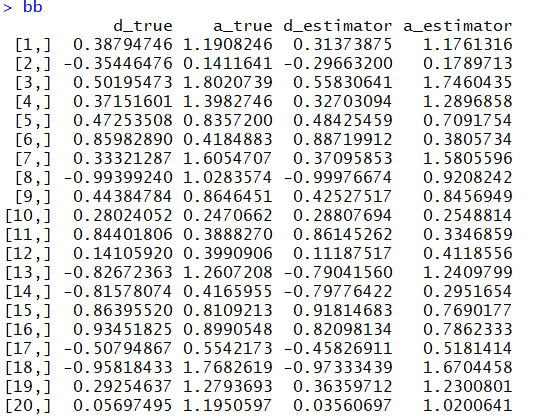关于simulation 的比较以及方法的各种性质的考量，应该多做一些replication，获得standard error以及mse等指标。这里没有再叙述。

https://zh.wikipedia.org/wiki/梯度下降法
https://zh.wikipedia.org/wiki/牛顿法
https://philchalmers.github.io/mirt/html/ltm_models_with_mirt.html
:  Baker, F. B., & Kim, S. H. (2004). Item response theory: Parameter estimation techniques. CRC Press.
:Chalmers, R. P. (2012). mirt: A multidimensional item response theory package for the R environment. Journal of Statistical Software, 48(6), 1-29.


展开全文em算法
• 算法分析应该怎么做？在许多情况下，分别运行两种...大部分算法都有影响运行时间的主要参数，这里是对求解问题规模的抽象度量，例如对于排序算法，它是要排序的元素数目。目的是通过简单的数学公式，尽可能地表达...


算法分析应该怎么做？在许多情况下，分别运行两种算法来解决同一问题是不理想的，面对这一困难，我们只好求助于数学工具，虽然我们无法对一个尚未完全实现的程序进行比较，但是通过数学分析，我们可以理解程序性能的大致轮廓，并且可以估计改进后的程序的效果。大部分算法都有影响运行时间的主要参数，这里是对求解问题规模的抽象度量，例如对于排序算法，它是要排序的元素数目。目的是通过简单的数学公式，尽可能地表达出程序的运行效率。功能增长对两种算法进行比较，我们不满足于简单地将其描述为“一种算法比另一种算法快”，而是希望能通过数学函数直观地了解两者的区别，特别是希望知道“一种算法比另一种算法快多少”。有些功能在算法分析中是非常常见的：…在一个程序中，如果大多数指令只运行一次或几次，与问题的大小无关，那么程序的运行时间是不变的。小高斯的算法是典型的常量时间。当问题规模增大时，程序的运行时间会变慢，因此可以认为程序的运行时间小于常数。尽管对数的底数影响函数值，但影响不大。考虑到计算机是进制的，所以它通常被取为底数值，这与数学(数学)略有不同。在适当的时候；在增加了一倍的时候,,只有微弱的增长；只有在增加了一倍的时候。若一种算法将一个大问题分解成几个小问题，每个小问题的运行时间都是常数，则我们认为该算法的运行时间是，典型的二分查找。该比率略高，当问题规模加倍时，运行时间比加倍时要短一些；当问题规模加倍时，程序运行时间会加倍。代价为 ntime的程序通常会处理程序的终止条件，例如，当判断一个数是否为质数时，边界值是质数的平方根，而非质数本身。通常称为线性时间，如果问题规模加倍，则程序运行时间也加倍。强制力达到的方法是线性时间，这类方法通常会有一个循环，结束于问题的规模。如果运行时间比问题大小增加一倍多一点，那么只需说明程序正在运行的时间。如果发生这种情况；如果 N=2048，不要把一个大问题分解成几个小问题，它们可以在一定的时间内运行，而要区分一下，是否需要合并这些小问题，如果合并，就是这样。大部分归并问题的运行时间可以简单地考虑。若问题的规模加倍，运行时间加倍；若问题的规模加倍，运行时间加倍。若问题的规模加倍，运行时间加倍；若问题的规模加倍，运行时间加倍。确实非常重要的增长假如；加倍努力，对于复杂问题，蛮力法一般都有这个尺度，这类算法一般不适用于实际问题。让我们看看这些功能的增长曲线，如图1所示。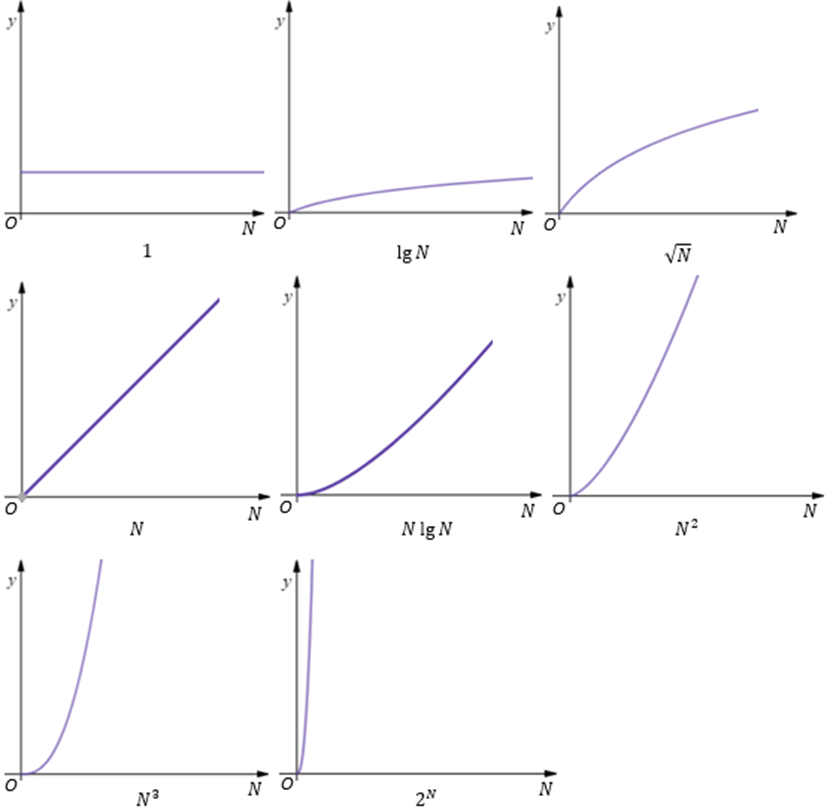`
展开全文• 改变里面参数，并分析其对相位估计的影响。 主要原理介绍 基本信道 在通信系统中，对信道的研究有着重要的作用。信道的作用是把携有信息的信号（电的或光的）从它的输入端传递到输出端，因此，它的最...
• 机器学习中的数学 觉得有用的话,欢迎一起讨论相互学习~Follow Me 原创文章,如需转载请...我们现在的主要目的是求1式的最大值,其中1式通过贝叶斯公式能转换为2式的形式,其中2式的分母相同,我们只需要考虑分子即可-...
• 转自随机信号功率谱密度估计 一、实验目的 1．深入理解随机信号功率谱密度估计 ...经典谱估计的方法主要包括两种方法：周期图法和自相关法。 周期图法（直接法） 周期图法又称为直接法，它是...
• 机器学习中的数学觉得有用的话,欢迎一起讨论相互学习~Follow Me ...我们现在的主要目的是求1式的最大值,其中1式通过贝叶斯公式能转换为2式的形式,其中2式的分母相同,我们只需要考虑分子即可–即3式,机器学习
• 写这篇文章的目的：最近看了一篇删失数据中地理加权COX模型，COX模型中考虑加入患者地理位置信息。该文章主要是理论推导类问题文章，文章中算法对应R包还未发布。发布后，在模型中添加患者地理信息也是个不错点...
• 这一过程的主要目的是在某些可再现性或平稳性的假设下预测QoS。该方法无须用户事先为业务源建立相应的业务模型并向网络提交其业务模型参数，而是通过对业务流的统计特性进行实时测量来动态估计相应的QoS参数，克服了...
• p^{-}混合随机变量序列加权和的强大数律，谭成良，吴群英，由于一些线形统计的研究是基于随机变量序列加权和的随机样本，例如最小均方差估计，非参数回归函数估计等.本文的主要目的是建立 p
• 本文的主要目的是获得由第二种两种帕累托（MTP）分布的有限混合表示的异类总体参数的推论。 基于渐进式II型删失样本应用了恒定局部加速寿命测试。 通过数值求解模型参数的似然方程，可以得出所考虑参数的最大似然...
• 训练/开发、测试集 最好验证集合测试集来自同一分布。 在机器学习中验证集合测试集含义分别如下： 验证集：对学习出来模型，调整分类器的参数，如在...测试集的目的是对所选定网络系统进行无偏估计，如果你...
• 数字波束合成也称为空域滤波，主要是根据信号环境变化自适应地改变各阵元加权因子，在期望信号方向形成主波束，在干扰信号方向形成零陷，降低副瓣电平, 目的是在增强期望信号同时最大程度抑制无用干扰和...
• 数字波束合成也称为空域滤波，主要是根据信号环境变化自适应地改变各阵元加权因子，在期望信号方向形成主波束，在干扰信号方向形成零陷，降低副瓣电平, 目的是在增强期望信号同时最大程度抑制无用干扰和...
• 方法论的主要目的是找到一种解决方案，以处理许多沿海沿海地区的各种异地使用参数，从而以其所有复杂性对其进行描述，特别是利用最新的欧洲卫星数据（Sentinel-2）。 这种复杂性是由于生态条件的局部变化，也归因于...
• EM算法进行参数估计的主要思想是： 目的是给定观测变量数据和隐变量数据以及分布函数来估计模型参数，首先选择参数的初始值，计算在此参数下观测数据来自各种可能（模型）的概率，这一步实现了将隐变量显式化，求得...算法
• 本文的主要目的是分析不同湍流模型对桥墩冲刷坑的影响。 Flow-3D软件的使用符合目的。 这项研究的主要动机是通过对泥沙冲刷模型和浅水模型进行一些修改和调整来为Flow-3D软件做出贡献。 进行了湍流模型评估，该模型...
• 对此,结合Copula函数研究特征变量之间的相关性优化问题,设计基于D-vine Copula理论的贝叶斯分类器,主要目的是为了提高类条件概率密度估计的准确性.将变量的联合概率分布分解为一系列二元Copula函数与边缘概率密度...
• 其方法主要可以分为两种，一种是基于大气退化模型，利用神经网络对模型中的参数进行估计，早期方法大多数是基于这种思想；另一种是利用输入有雾图像，直接输出得到去雾后图像。目前最新去雾方法更倾向于后...图像去雾算法
• 目的：本研究的主要目的是比较三种统计模型，以评估医院死亡率与两个危险因素之间的关联性，即受试者的入院年龄和住院时间，并调整诊断相关组（DRG）的效果。 方法：我们使用几种SAS程序来量化DRG对医院死亡率变异...
• 该研究考察了小额信贷对民生的影响，本文的主要目的是调查和分析小额信贷对红海州居民生活的影响。 在方法论上，研究使用计量经济学和描述性统计分析方法。 使用回归方程。 我们检查了时间序列数据，这是许多研究和...
• 该工具的主要目的是在不久的将来探索COVID19病例的动态以及相关的卫生保健系统压力。 疫情受学校关闭，封锁等感染控制措施的影响。可以通过调整缓解参数将这些措施的效果包括在模拟中。 类似地，您可以在严重程度...